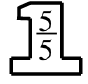### Home > CC2MN > Chapter 4 > Lesson 4.3.3 > Problem4-115

4-115.

Copy each expression and simplify it. Be sure to show the steps you use to get the answer.

1. $\frac{19}{20}-\frac{1}{4}$

Remember that

$\frac{1}{4}\cdot$$=\frac{5}{20}$

$\frac{14}{20} = \frac{7}{10}$

1. $\frac{22}{25}-\frac{7}{10}$

One way to start is to change both so that they have $100$ in the denominator.

$\frac{22}{25}\cdot \frac{4}{4} = \frac{88}{100}$

$\frac{7}{10}\cdot\frac{?}{?} = ?$

$\frac{9}{50}$

1. $\frac{9}{32}+\frac{7}{8}$

See part (a).